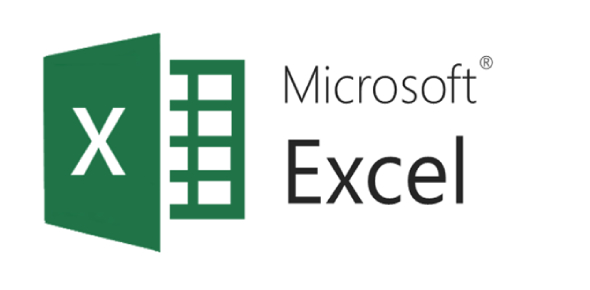# MS Excel Basics Quiz Questions And Answers

14 Questions | Attempts: 12565
ShareSettings.

• 1.
_______, ________ and _______ are what you see when you open Excel
• A.

Columns, rows, and charts

• B.

Columns, rows, and formulas

• C.

Columns, rows, and cells

• D.

Non of the above

• 2.
Ribbon is MS Excel is
• A.

Commands organized in small related groups is called Ribbon

• B.

The band at the top of the Excel 2007 window is the Ribbon

• C.

All of the above

• D.

Non of the above

• 3.
When you start Excel, you open a file that's called a workbook. Each new workbook comes with_____________ worksheet(s), like pages in a document. You enter data into the worksheets. (Worksheets are sometimes called spreadsheets.)
• A.

One

• B.

Two

• C.

Three

• D.

Four

• 4.
___________ are where you get down to business and enter data in a worksheet.
• A.

Cells

• B.

Fields

• C.

Tables

• D.

None of the above

• 5.
In a new worksheet, you must start by typing in cell A1.
• A.

True

• B.

False

• 6.
You can use Excel to create budgets, to work with taxes, or to record student grades
• A.

True

• B.

False

• 7.
You can enter two basic kinds of data into worksheet cells:
• A.

Numbers and text

• B.

Numbers and figures

• C.

Text and charts

• D.

None of the above

• 8.
To enter a date in a cell, you should use a slash or a hyphen to separate the parts: 7/16/2009 or 16-July-2009. Excel will recognize this as a text
• A.

True

• B.

False

• 9.
You learned in the practice that ###### means:
• A.

You've entered a number wrong

• B.

You've misspelled something

• C.

The cell is not wide enough

• 10.
What do you type into an empty cell to start a formula?
• A.

An equal sign (=)

• B.

A plus sign (+)

• C.

Number

• D.

Fx

• 11.
A formula result is in cell C6. You wonder how you got the result. To see the formula, you:
• A.

Click in cell C6, and then press CTRL+SHIFT.

• B.

Click in cell C6, and then press CTRL+SHIFT.

• C.

Click in cell C6.

• D.

Non of the above

• 12.
To divide 853 by 16 in a formula in Excel, you would use what math operator?
• A.

+

• B.

=

• C.

%

• D.

/

• 13.
Cell Reference:A10,A20Refer to values in
• A.

The cell in column A and row 10

• B.

Cell A10 and cell A20

• C.

The range of cells in column A and rows 10 through 20

• D.

The range of cells in row 15 and columns B through E

• 14.
A10:A20Refer to values in
• A.

The cell in column A and row 10

• B.

Cell A10 and cell A20

• C.

The range of cells in column A and rows 10 through 20

• D.

The range of cells in row 15 and columns B through E

## Related TopicsBack to top
×

Wait!
Here's an interesting quiz for you.